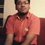# Digit Sum Inequality

Inspired by [http://en.wikipedia.org/wiki/Digit_sum] "digit sums for binary being on average smaller than those for any other base." Here's my attempt at the proposition.

if R is the radix, & N is the given integer value, the number of digits n required on radix R to represent N = [ln(N*R)/lnR], yes [] is the greatest integer or the floor function....

Clearly, N = A0 + A1R + A2Rsq + A3Rcube + ...... An(R^n) = Sum(Ai*R^i) & the digit sum DS = A0 + A1 + A2 + A3 + ...... +An = Sum(Ai)

we know CS inequality..., check the special case #1 of this link for reference. [http://en.wikipedia.org/wiki/Cauchy–Schwarz_inequality]

put Ai = ai, & R^i = bi... we get, [Sum(AiR^i)]sq <= Sum(Aisq)Sum(R^i sq)

Look closely, its... Nsq <= Sum(Aisq)Sum(R^i sq)... & Sum(R^i sq) is a GP..., we get Nsq <= Sum(Aisq)[(Rsq^n -1)/(Rsq-1)]...

Now we also know PowerMean inequality [https://www.artofproblemsolving.com/Wiki/index.php/PowerMeanInequality] according to which... sqrt[Avg(Aisq)]>=Avg(Ai).... write avg(Ai) = (1/n)*sum(Ai)..., mind you sum(Ai) is DS. Now substitute this into the Nsq inequality... we will get....

Nsq <= [(DS)sq/n][(Rsq^n -1)/(Rsq-1)]... &..., DS >= Nsqrt[n*(Rsq-1)/(Rsq^n-1)]

You can see that for any N, if we increase R from 2, the curve agrees with the proposition. With equality holding only for n=1, which makes sense, if R>N, then N can be represented on R in a single digit & DS would = N.Note by Jayant Kumar
7 years, 1 month ago

This discussion board is a place to discuss our Daily Challenges and the math and science related to those challenges. Explanations are more than just a solution — they should explain the steps and thinking strategies that you used to obtain the solution. Comments should further the discussion of math and science.

When posting on Brilliant:

• Use the emojis to react to an explanation, whether you're congratulating a job well done , or just really confused .
• Ask specific questions about the challenge or the steps in somebody's explanation. Well-posed questions can add a lot to the discussion, but posting "I don't understand!" doesn't help anyone.
• Try to contribute something new to the discussion, whether it is an extension, generalization or other idea related to the challenge.

MarkdownAppears as
*italics* or _italics_ italics
**bold** or __bold__ bold
- bulleted- list
• bulleted
• list
1. numbered2. list
1. numbered
2. list
Note: you must add a full line of space before and after lists for them to show up correctly
paragraph 1paragraph 2

paragraph 1

paragraph 2

[example link](https://brilliant.org)example link
> This is a quote
This is a quote
    # I indented these lines
# 4 spaces, and now they show
# up as a code block.

print "hello world"
# I indented these lines
# 4 spaces, and now they show
# up as a code block.

print "hello world"
MathAppears as
Remember to wrap math in $$ ... $$ or $ ... $ to ensure proper formatting.
2 \times 3 $2 \times 3$
2^{34} $2^{34}$
a_{i-1} $a_{i-1}$
\frac{2}{3} $\frac{2}{3}$
\sqrt{2} $\sqrt{2}$
\sum_{i=1}^3 $\sum_{i=1}^3$
\sin \theta $\sin \theta$
\boxed{123} $\boxed{123}$